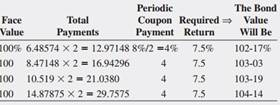### Create an Account

Home / Questions / Using a Spreadsheet to Calculate Bond Values What is the bond quote for a \$1000 face value...

# Using a Spreadsheet to Calculate Bond Values What is the bond quote for a \$1000 face value bond with an 8 percent coupon rate paid semiannually and a required return of 7 5 percent if the bond is

Using a Spreadsheet to Calculate Bond Values: What is the bond quote for a \$1,000 face value bond with an 8 percent coupon rate (paid semiannually) and a required return of 7.5 percent if the bond is 6.48574, 8.47148, 10.519, and 14.87875 years from maturity?May 18 2020 View more View LessSubscribe To Get Solution